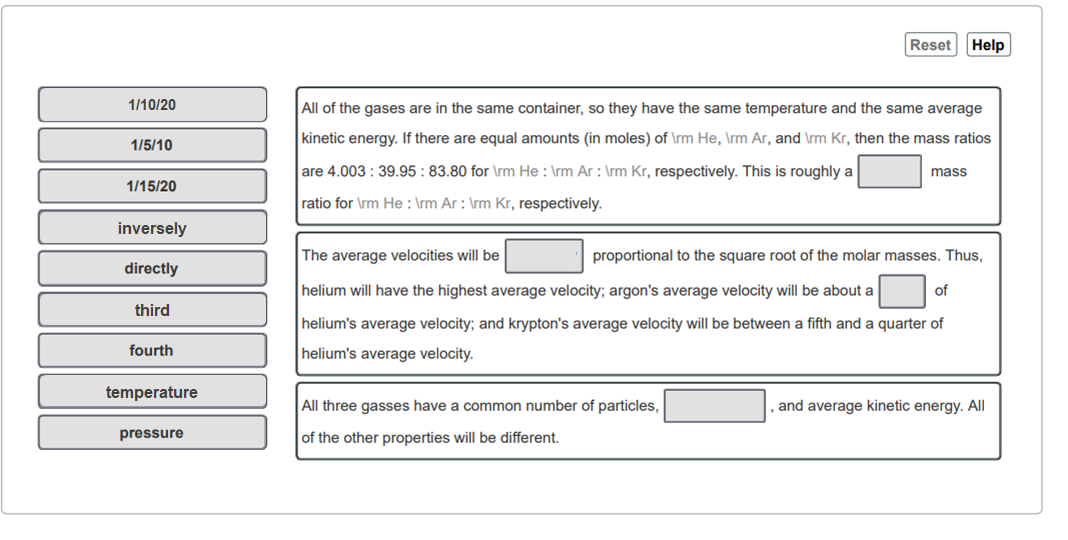# Problem: A box contains equal amounts of helium, argon, and krypton (all gases) at 25 C. Describe the temperatures, masses, average velocities, and average kinetic energy of the three kinds of gas in the mixture. What do they have in common? What are the differences? How are these properties related? Match the words in the left column to the appropriate blanks in the sentences on the right .

###### FREE Expert Solution

Mass ratios:

0.10 or 1/10

~0.50 or 1/2 = 10/20

Average velocity (related to molecular speed) → inversely proportional to square root of molar mass:

~ 0.33 or 1/3 (one third)###### Problem Details

A box contains equal amounts of helium, argon, and krypton (all gases) at 25 C. Describe the temperatures, masses, average velocities, and average kinetic energy of the three kinds of gas in the mixture. What do they have in common? What are the differences? How are these properties related?

Match the words in the left column to the appropriate blanks in the sentences on the right .